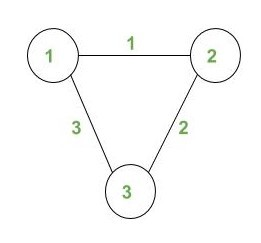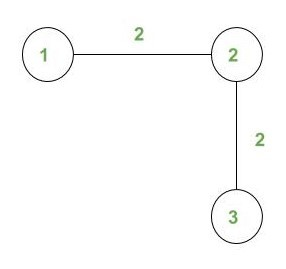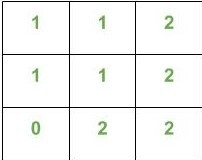# Count the number of walks of length N where cost of each walk is equal to a given number

• Last Updated : 05 Aug, 2022

Given a weighted undirected graph, Length of walks N and Cost X. The task is to count the number of different walks W of length N such that Cost(W) = X.
We define the cost of a walk W, as the maximum over the weights of the edges along the walk.
The nodes are numbered from 1 to n. The graph does not contain any multiple edges or self-loops.

Examples:

Input:N = 4, X = 2
Output: 10
Explanation :
A walk W on the graph is a sequence of vertices (with repetitions of vertices and edges allowed) such that every adjacent pair of vertices in the sequence is an edge of the graph.
For X = 2, all possible 10 walks are listed below :

1. 1 -> 2 -> 1 -> 2 -> 3
2. 1 -> 2 -> 3 -> 2 -> 1
3. 1 -> 2 -> 3 -> 2 -> 3
4. 2 -> 1 -> 2 -> 3 -> 2
5. 2 -> 3 -> 2 -> 1 -> 2
6. 2 -> 3 -> 2 -> 3 -> 2
7. 3 -> 2 -> 1 -> 2 -> 1
8. 3 -> 2 -> 1 -> 2 -> 3
9. 3 -> 2 -> 3 -> 2 -> 1
10. 3 -> 2 -> 3 -> 2 -> 3

Input:N = 4, X = 2
Output: 12

• The idea is to precalculate the no. of walks of length N for each vertex of all possible cost and store them in a 2-D matrix.Let us call this matrix as B.These values can be calculated by running DFS on the given undirected graph.
For example,The given snapshot of matrix B shows the values stored in it. here B(i, j) means no. of walks of length N from vertex i having cost of walk j.

• We maintain a 1-D array Maxedge in which we keep the cost of walk of length N. We call the same function when the length of walk is less than N and there is some cost X associated with edge(u, v).
We put a base condition for length == N for which we update the array B and return the call.
• After calculating the matrix B we simply count the total no of walks by adding the no of walks of all the vertex having cost = x.

Ans += B[i][x];
Here i ranges from 1 to n where n is the no of vertices.

Below is the implementation of the above approach

## C++

 `// C++ program to count the number of walks``// of length N where cost of each walk is``// equal to k``#include ``using` `namespace` `std;``int` `G = {0};``int` `Maxedge = {0};``int` `B = {0};``int` `l = 0, n, m;` `// Function return total``// walk of length N``int` `TotalWalks(``int` `cost)``{``     ``int` `ans=0;``    ` `    ``// Add values of all``    ``// node with cost X``     ``for``(``int` `i=1;i<=n;i++)``     ``{``        ``ans+=B[i][cost];``     ``}``    ` `     ``return` `ans;``}`  `// Function to precompute array B``// meantioned above``void` `DFS(``int` `u, ``int` `v,``int` `len)``{``    ``// Base condition``    ``if` `(l == len)             ``    ``{``        ``// Updating the matrix B when``        ``// we get a walk of length N.``        ``B[u][ Maxedge[len]]++;``        ``return` `;``    ``}``    ``for` `(``int` `i = 1; i <= n; i++)``    ``{``        ``if` `(G[v][i] !=0)``        ``{``            ``// Incrementing the length``            ``// of the walk``            ``l++;``            ` `            ``// Updating the cost of the walk``            ``Maxedge[l] = max(Maxedge[l - 1],``                             ``G[v][i]);``            ``DFS(u, i, len);``            ``l--;``        ``}``    ``}``}` `// Function to calculate total``// number of walks of length N``void` `NumberOfWalks(``int` `cost,``int` `len)``{``    ``for` `(``int` `i = 1; i <= n; i++)``    ``{``        ``// Calling the function DFS``        ``DFS(i, i, len); ``    ``}``    ` `    ``int` `ans = TotalWalks(cost);``    ` `    ``// Print the answer``    ``cout<< ans << endl;``}` `// Driver code``int` `main()``{``    ``int` `Cost = 2;``    ``n = 3, m = 3;``    ``int` `length = 4;``    ` `    ``// Create a graph given in``    ``// the above diagram``    ``G = 1;``    ``G = 1;``    ``G = 2;``    ``G = 2;``    ``G = 3;``    ``G = 3;``    ` `    ``NumberOfWalks(Cost, length) ;``}`

## Java

 `// Java program to count the number of walks``// of length N where cost of each walk is``// equal to k``import` `java.util.*;` `class` `GFG{``    ` `static` `int` `[][]G = ``new` `int``[``250``][``250``];``static` `int` `[]Maxedge = ``new` `int``[``250``];``static` `int` `[][]B = ``new` `int``[``250``][``250``];``static` `int` `l = ``0``, n, m;` `// Function return total``// walk of length N``static` `int` `TotalWalks(``int` `cost)``{``    ``int` `ans = ``0``;``    ` `    ``// Add values of all``    ``// node with cost X``    ``for``(``int` `i = ``1``; i <= n; i++)``    ``{``        ``ans += B[i][cost];``    ``}``    ` `    ``return` `ans;``}` `// Function to precompute array B``// meantioned above``static` `void` `DFS(``int` `u, ``int` `v, ``int` `len)``{``    ``// Base condition``    ``if` `(l == len)            ``    ``{``        ` `        ``// Updating the matrix B when``        ``// we get a walk of length N.``        ``B[u][ Maxedge[len]]++;``        ``return``;``    ``}``    ` `    ``for` `(``int` `i = ``1``; i <= n; i++)``    ``{``        ``if` `(G[v][i] !=``0``)``        ``{``            ` `            ``// Incrementing the length``            ``// of the walk``            ``l++;``            ` `            ``// Updating the cost of the walk``            ``Maxedge[l] = Math.max(Maxedge[l - ``1``],``                                        ``G[v][i]);``            ``DFS(u, i, len);``            ``l--;``        ``}``    ``}``}` `// Function to calculate total``// number of walks of length N``static` `void` `NumberOfWalks(``int` `cost,``int` `len)``{``    ``for``(``int` `i = ``1``; i <= n; i++)``    ``{` `       ``// Calling the function DFS``       ``DFS(i, i, len);``    ``}``    ` `    ``int` `ans = TotalWalks(cost);``    ` `    ``// Print the answer``    ``System.out.print(ans + ``"\n"``);``}` `// Driver code``public` `static` `void` `main(String[] args)``{``    ``int` `Cost = ``2``;``    ``n = ``3``; m = ``3``;``    ``int` `length = ``4``;``    ` `    ``// Create a graph given in``    ``// the above diagram``    ``G[``1``][``2``] = ``1``;``    ``G[``2``][``1``] = ``1``;``    ``G[``2``][``3``] = ``2``;``    ``G[``3``][``2``] = ``2``;``    ``G[``1``][``3``] = ``3``;``    ``G[``3``][``1``] = ``3``;``    ` `    ``NumberOfWalks(Cost, length);``}``}` `// This code is contributed by 29AjayKumar`

## Python3

 `# Python3 program to count the number of walks``# of length N where cost of each walk is``# equal to k``G ``=` `[[``0` `for` `i ``in` `range``(``250``)]``        ``for` `j ``in` `range``(``250``)]``Maxedge ``=` `[``0` `for` `i ``in` `range``(``250``)]``B ``=` `[[``0` `for` `i ``in` `range``(``250``)]``        ``for` `j ``in` `range``(``250``)]``l ``=` `0``n ``=` `0``m ``=` `0`` ` `# Function return total``# walk of length N``def` `TotalWalks(cost):` `    ``ans ``=` `0``     ` `    ``# Add values of all``    ``# node with cost X``    ``for` `i ``in` `range``(``1``, n ``+` `1``):``        ``ans ``+``=` `B[i][cost]``     ` `    ``return` `ans` `# Function to precompute array B``# meantioned above``def` `DFS(u, v, ``len``):``    ` `    ``global` `l``    ` `    ``# Base condition``    ``if` `(l ``=``=` `len``):``    ` `        ``# Updating the matrix B when``        ``# we get a walk of length N.``        ``B[u][ Maxedge[``len``]] ``+``=` `1``        ``return``    ` `    ``for` `i ``in` `range``(``1``, n ``+` `1``):``        ``if` `(G[v][i] !``=` `0``):``        ` `            ``# Incrementing the length``            ``# of the walk``            ``l ``+``=` `1``             ` `            ``# Updating the cost of the walk``            ``Maxedge[l] ``=` `max``(Maxedge[l ``-` `1``], G[v][i])``            ``DFS(u, i, ``len``)``            ``l ``-``=` `1``        ` `# Function to calculate total``# number of walks of length N``def` `NumberOfWalks(cost, ``len``):``    ` `    ``for` `i ``in` `range``(``1``, n ``+` `1``):``    ` `        ``# Calling the function DFS``        ``DFS(i, i, ``len``) ``    ` `    ``ans ``=` `TotalWalks(cost)``     ` `    ``# Print the answer``    ``print``(ans)``    ` `# Driver code``if` `__name__``=``=``'__main__'``:` `    ``Cost ``=` `2``    ``n ``=` `3``    ``m ``=` `3``    ``length ``=` `4``     ` `    ``# Create a graph given in``    ``# the above diagram``    ``G[``1``][``2``] ``=` `1``    ``G[``2``][``1``] ``=` `1``    ``G[``2``][``3``] ``=` `2``    ``G[``3``][``2``] ``=` `2``    ``G[``1``][``3``] ``=` `3``    ``G[``3``][``1``] ``=` `3``     ` `    ``NumberOfWalks(Cost, length)` `# This code is contributed by rutvik_56`

## C#

 `// C# program to count the number of walks``// of length N where cost of each walk is``// equal to k``using` `System;` `class` `GFG{``    ` `static` `int` `[,]G = ``new` `int``[250, 250];``static` `int` `[]Maxedge = ``new` `int``;``static` `int` `[,]B = ``new` `int``[250, 250];``static` `int` `l = 0, n;` `// Function return total``// walk of length N``static` `int` `TotalWalks(``int` `cost)``{``    ``int` `ans = 0;``    ` `    ``// Add values of all``    ``// node with cost X``    ``for``(``int` `i = 1; i <= n; i++)``    ``{``       ``ans += B[i, cost];``    ``}``    ``return` `ans;``}` `// Function to precompute array B``// meantioned above``static` `void` `DFS(``int` `u, ``int` `v, ``int` `len)``{` `    ``// Base condition``    ``if` `(l == len)            ``    ``{``        ` `        ``// Updating the matrix B when``        ``// we get a walk of length N.``        ``B[u, Maxedge[len]]++;``        ``return``;``    ``}``    ` `    ``for``(``int` `i = 1; i <= n; i++)``    ``{``       ``if` `(G[v, i] != 0)``       ``{``           ` `           ``// Incrementing the length``           ``// of the walk``           ``l++;``           ` `           ``// Updating the cost of the walk``           ``Maxedge[l] = Math.Max(Maxedge[l - 1],``                                       ``G[v, i]);``           ``DFS(u, i, len);``           ``l--;``       ``}``    ``}``}` `// Function to calculate total``// number of walks of length N``static` `void` `NumberOfWalks(``int` `cost, ``int` `len)``{``    ``for``(``int` `i = 1; i <= n; i++)``    ``{``       ` `       ``// Calling the function DFS``       ``DFS(i, i, len);``    ``}``    ` `    ``int` `ans = TotalWalks(cost);``    ` `    ``// Print the answer``    ``Console.Write(ans + ``"\n"``);``}` `// Driver code``public` `static` `void` `Main(String[] args)``{``    ``int` `Cost = 2;``    ``n = 3;``    ``int` `length = 4;``    ` `    ``// Create a graph given in``    ``// the above diagram``    ``G[1, 2] = 1;``    ``G[2, 1] = 1;``    ``G[2, 3] = 2;``    ``G[3, 2] = 2;``    ``G[1, 3] = 3;``    ``G[3, 1] = 3;``    ` `    ``NumberOfWalks(Cost, length);``}``}` `// This code is contributed by gauravrajput1`

## Javascript

 ``

Output:

`10`

My Personal Notes arrow_drop_up